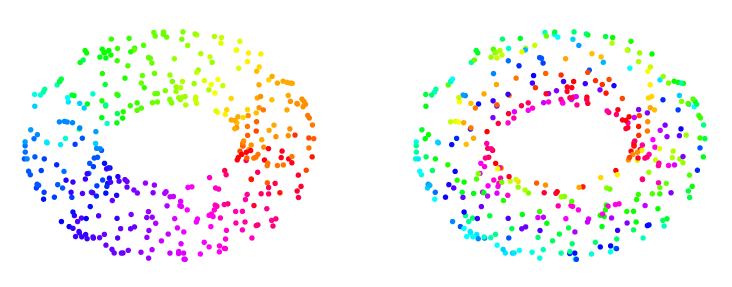## Persistent Cohomology and Circular Coordinates

 Discrete and Computational Geometry, vol. 45, pages 737-759, 2011.DCG DOI: 10.1007/s00454-011-9344-x
Abstract
Nonlinear dimensionality reduction (NLDR) algorithms such as Isomap, LLE and Laplacian Eigenmaps address the problem of representing high-dimensional nonlinear data in terms of low-dimensional coordinates which represent the intrinsic structure of the data. This paradigm incorporates the assumption that real-valued coordinates provide a rich enough class of functions to represent the data faithfully and efficiently. On the other hand, there are simple structures which challenge this assumption: the circle, for example, is one-dimensional but its faithful representation requires two real coordinates. In this work, we present a strategy for constructing circle-valued functions on a statistical data set. We develop a machinery of persistent cohomology to identify candidates for significant circle-structures in the data, and we use harmonic smoothing and integration to obtain the circle-valued coordinate functions themselves. We suggest that this enriched class of coordinate functions permits a precise NLDR analysis of a broader range of realistic data sets.